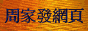# 廣義量詞系列：量詞的邏輯性質

## 2. 類可歸約性

### 2.1 基本概念

Some boy sang.
some(BOY)(SING)
∃x ∈ U (x ∈ BOY ∧ x ∈ SING)
Every boy sang.
every(BOY)(SING)
∀x ∈ U (x ∈ BOY ⇒ x ∈ SING)

some(U)(BOY ∩ SING)     (1)

∀x ∈ U (x ~∈ BOY ∨ x ∈ SING)

every(U)(~BOY ∪ SING)     (2)

Q(A)(B) ⇔ Q(U)(Bool(A, B))     (3)

### 2.2 主要定理

Keenan在Quantification in English is Inherently Sortal一文中研究了「類可歸約性」的問題，提出了一條完全確定「類可歸約限定詞」的定理。為方便以下的證明，這裡首先引入另一條定理。

 定理1： (i) Q ∈ INT當且僅當對任何A、B，均有Q(A)(B) ⇔ Q(U)(A ∩ B)。 (ii) Q ∈ RC-INT當且僅當對任何A、B，均有Q(A)(B) ⇔ Q(U)(~A ∪ B)。 (iii) 如對任何A、B，均有Q(A)(B) ⇔ Q(U)(~A ∪ ~B)，則Q ∈ INT。 (iv) 如對任何A、B，均有Q(A)(B) ⇔ Q(U)(A − B)，則Q ∈ RC-INT。

 Bool1(A, B) = U Bool2(A, B) = Φ Bool3(A, B) = A Bool4(A, B) = ~A Bool5(A, B) = B Bool6(A, B) = ~B Bool7(A, B) = A ∩ B Bool8(A, B) = ~A ∪ ~B Bool9(A, B) = A − B Bool10(A, B) = ~A ∪ B Bool11(A, B) = B − A Bool12(A, B) = A ∪ ~B Bool13(A, B) = ~A ∩ ~B Bool14(A, B) = A ∪ B Bool15(A, B) = (A − B) ∪ (B − A) Bool16(A, B) = (A ∩ B) ∪ (~A ∩ ~B)

### 2.3 例證

「定理2」告訴我們，「相交限定詞」和「右補相交限定詞」是「類可歸約」的。如果我們把「定理1(i)和(ii)」分別與(1)和(2)比較，我們將會看到，「相交限定詞」和「右補相交限定詞」在進行「類歸約」後所得的「布爾組合」分別與"some"和"every"進行「類歸約」後所得的「布爾組合」具有相同的形式(註2)。我們可以根據此一關係得到一些有效推理，首先看「相交限定詞」的例子：

Some boy is singing. ⇔ Some individual is a boy who is singing.
No boy except John is singing. ⇔ No individual except John is a boy who is singing.
Infinitely many points are contained in the circle.
⇔ Infinitely many individuals are points contained in the circle.

Every boy is singing. ⇔ Every individual is such that if he is a boy then he is singing.
Every boy except John is singing. ⇔ Every individual except John is such that if he is a boy then he is singing.
All but finitely many points are contained in the circle.
⇔ All but finitely many individuals are such that if it is a point then it is contained in the circle.

「定理2」也告訴我們，其他限定詞都是「類固定」的。在自然語言中，最重要的「類固定限定詞」包括那些包含「預設」的限定詞，例如"the"、"John's"、"both"、"neither"等以及某些「右比例限定詞」(記作PROPORTr)，例如"most"、"(less than q)"、"(no except q)"以及「右補右比例限定詞」(記作RC-PROPORTr)，例如"(all except q)"等(其中q代表小於1的分數)。上述這些限定詞都不能進行「類歸約」，例如以下推導便是無效的：

Most boys are singing. ~⇔ Most individuals are boys who are singing.

## 3. 存在性

### 3.1 存在句

There are some students playing in the park.

some(STUDENT ∩ {x: x is playing in the park})(U)

There is no boy except John who knows the secret.
There were at least seven attendants in the meeting.
?There is every family member to support you.
?There are most students playing.

### 3.2 主要定理

Keenan在A Semantic Definition of "Indefinite NP"一文中研究了「存在句」的限定詞問題，他提出「存在性」(Existentiality)的概念，即限定詞Q是「存在」(記作EXIST)的當且僅當對任何A、B均有

Q(A)(B) ⇔ Q(A ∩ B)(U)     (4)

Keenan認為形如「There + be + Q + A + B」的「存在句」在語義上應被解釋成等同於「Q(A)(B)」(註3)。當Q滿足定義(4)的條件時，這類「存在句」在邏輯上等價於Q(A ∩ B)(U)。這裡U作為一個謂詞性論元，可以被解讀成論域中所有元素都滿足的謂語，例如"exist"。換句話說，根據上述定義，上一小節舉出的合語法「存在句」都滿足以下等價關係：

 There is no boy except John who knows the secret. ⇔ No boy except John knows the secret. ⇔ Except for John, no boy who knows the secret exists.
 There were at least seven attendants in the meeting. ⇔ At least seven attendants were in the meeting. ⇔ At least seven attendants who were in the meeting existed.

Keenan認為，即使是不合語法的「存在句」也應被解釋成等同於「Q(A)(B)」。它們跟合語法「存在句」的區別僅在於在邏輯上並不等價於一個以"exist"作為謂語的量化句，例如

 ?There is every family member to support you. ⇔ Every family member is to support you. ~⇔ Every family member who is to support you exists.
 ?There are most students playing. ⇔ Most students are playing. ~⇔ Most students who are playing exist.

Keenan並指出，並非所有「存在限定詞」都可出現於「存在句」中。因此，「存在性」只是可出現於「存在句」的必要條件而非充分條件。舉例說，"(either all or else not all)"便具有「存在性」，因為這個限定詞涵蓋所有可能性，實際等同於"1"，而1 ∈ INT (參見註1)；但這個限定詞在結構上類似限定詞"all"，而後者不能出現於「存在句」中。

### 3.3 概念推廣

Q(A) ⇔ Qrel(U)(A)

Q(A) ⇔ Qrel(A)(U)     (5)

There is nothing inside the box.
There is somebody living here.
?There is everything you need.
?There was everybody except Mary in the ball.

Q(A, B)(C) ⇔ Q(A ∩ C, B ∩ C)(U)     (6)

There are more boys than girls participating in the game.
There are exactly as many boys as girls participating in the game.

## 4. 一階可定義性

### 4.1 基本概念

「一階邏輯」(First Order Logic)(簡稱FO，這裡是指「帶等詞的一階謂詞邏輯」)是在現代數理邏輯中最受重視的邏輯系統，因為它較為簡單，而且具有許多良好的元邏輯性質。不過，由於「一階邏輯」的「邏輯常項」只包括邏輯聯結詞(~、∧、∨、⇒、⇔)、兩個量詞(∀、∃)和一個等詞(=)，它的表達力十分有限。廣義量詞理論中的一個重要課題就是研究自然語言中哪些量詞可以用「一階語句」(First Order Sentence)來表達，所謂「一階語句」，就是指使用上述「邏輯常項」以及其他常項、變項，依照「一階邏輯」的形成規則，經有窮步驟形成的不含自由變項的合式公式(詳細內容請參閱《廣義量詞系列：現代推理模式》)。

p在M中真 ⇔ Q(A)(B)在M中真

∃x∃y∃z (x ≠ y ∧ y ≠ z ∧ z ≠ x ∧ A(x) ∧ A(y) ∧ A(z) ∧ B(x) ∧ B(y) ∧ B(z))
∀x∀y∀z ((A(x) ∧ A(y) ∧ A(z) ∧ B(x) ∧ B(y) ∧ B(z)) ⇒ (x = y ∨ y = z ∨ z = x))

∃x∀y (A(x) ∧ (A(y) ⇒ y = x) ∧ B(x))

### 4.2 與左單調性的關係

(at most n)(A)(B) ∨ (at most m)(A)(~B)     (7)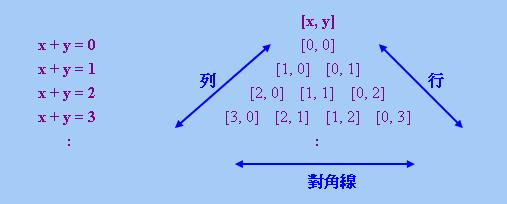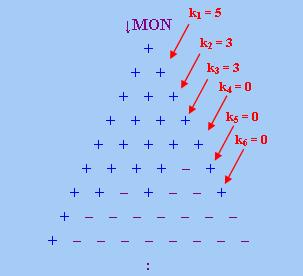no(A)(B) ∨ (at most 5)(A)(~B)
(at most 1)(A)(B) ∨ (at most 3)(A)(~B)
(at most 3)(A)(B) ∨ no(A)(~B)
(at most 6)(A)(B)

↓MON ⊆ FO-D     (8)

↑MON ⊆ FO-D     (9)

### 4.3 與左連續性的關係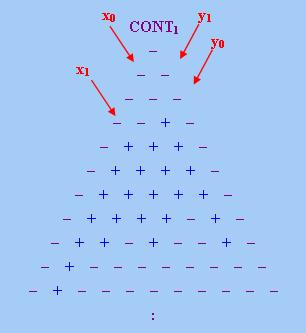Q'[x, y] ⇔ Q[x, y] ∨ (x0 ≤ x ≤ x1 ∧ y1 ≤ y ≤ y0)

no(A)(B) ∨ (at most 5)(A)(~B)

(exactly 1)(A)(B) ∨ (between 1 and 6)(A)(~B)

((exactly 1)(A)(B) ∨ (between 1 and 6)(A)(~B))
∧ ((between 1 and 2)(A)(B) ∨ (between 1 and 4)(A)(~B))
∧ ((between 1 and 4)(A)(B) ∨ (exactly 1)(A)(~B))
∧ (between 1 and 7)(A)(B)
∧ ~((exactly 1)(A)(~B) ∧ (exactly 1)(A)(B))
∧ ~((exactly 2)(A)(~B) ∧ (exactly 1)(A)(B))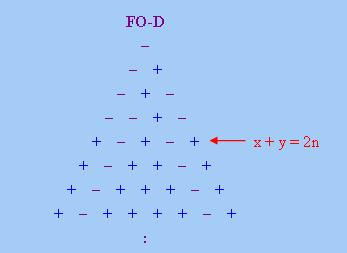((at least 2)(A)(B) ∧ (at least 2)(A)(~B))
∨ (no(A)(B) ∧ (at least 4)(A)(~B))
∨ ((at least 4)(A)(B) ∧ no(A)(~B))
∨ (no(A)(~B) ∧ (exactly 1)(A)(B))
∨ ((exactly 1)(A)(~B) ∧ (exactly 1)(A)(B))
∨ ((exactly 1)(A)(~B) ∧ (exactly 2)(A)(B))

「定理5」和「定理6」告訴我們，「一階可定義性」與「左連續性」有密切關係。可是，這兩條定理對於證明某些限定詞的「一階不可定義性」卻不甚方便。舉例說，雖然我們知道"(an even number of)"、"(an odd number of)"以及很多「右比例限定詞」和「右補右比例限定詞」不是「左連續」的，但要證明它們不能表達為有限個「左連續限定詞」的析取，卻似乎不是簡單的事。對於定義於無限論域上的限定詞(例如"(infinitely many)")，這兩條定理就更加是無能為力。不過，我們還有其他方法證明上述這些限定詞的「一階不可定義性」，這將留待下文再作介紹。

### 4.4 類可歸約性、存在性與一階可定義性的關係

EXIST ⊂ SR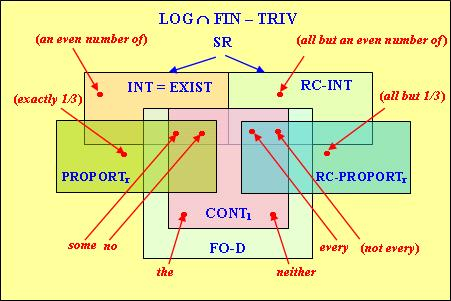## 5. 一階(量詞)可定義性

### 5.1 一階邏輯的推廣

∃f (f ∈ (A − B) → (A ∩ B) ∧ INJECTIVE(f) ∧ ~SURJECTIVE(f))

||most x (A(x), B(x))|| = 1當且僅當|A ∩ B| > |A − B|，其中A = {a: ||A[a/x]|| = 1}，B = {a: ||B[a/x]|| = 1}。

p在M中真 ⇔ Q(A)(B)在M中真

 定理8： 設q、q'、q''為量詞序列， (i) FO(q) ≤ FO(q, q') (ii) 設FO(q) ≤ FO(q')，若對q''中任何成員Q''，Q'' ∈ FO(q)-D，則Q'' ∈ FO(q')-D (iii) 設FO(q) ≤ FO(q')，若對q'中任何成員Q'，Q' ∈ FO(q'')-D，則對q中任何成員Q，Q ∈ FO(q'')-D

### 5.2 可定義性的證明

FO(q, q') ≤ FO(q)當且僅當對序列{q, q'}中任何成員Q*而言，都有Q* ∈ FO(q)-D。

「定理9」告訴我們，如果我們把在FO(q)可定義的量詞加入FO(q)中，所得邏輯系統等價於原來的FO(q)。利用此一定理以及前面有關「一階可定義性」的結果，我們馬上得到一些結果，例如：

FO((at least 3), (at most 2), the) ≡ FO

 定理10： (i) FO(Q0) ≤ FO(I) ≤ FO(MO) (ii) FO(QR) ≤ FO(most) ≤ FO(MO) (iii) FO(MO) ≤ FO(QH) (iv) FO(Qeven) ≤ FO(QH)

Q0(A) ⇔ A有無窮多個元素
I(A)(B) ⇔ |A| = |B|
MO(A)(B) ⇔ |A| > |B|
QR(A) ⇔ |A| > |~A|
QH(R) ⇔ ∃f∃g∀x∀y (R(x, f(x), y, g(y))
Qeven(A) ⇔ |A|為偶數

 Q0(A) ⇔ ∃x (A(x) ∧ |A| = |A − {x}|) ⇔ ∃x (A(x) ∧ |{y: A(y)}| = |{y: A(y) ∧ y ≠ x}|) ⇔ ∃x (A(x) ∧ Iy (A(y), A(y) ∧ y ≠ x))

(i)的後半部可證明如下：

 I(A)(B) ⇔ |A| ≤ |B| ∧ |B| ≤ |A| ⇔ ~MOx (A(x), B(x)) ∧ ~MOx (B(x), A(x))

(ii)的前半部可證明如下：

 QR(A) ⇔ |A| > |~A| ⇔ most(U)(A) ⇔ most({x: x = x})({x: A(x)}) ⇔ most x (x = x, A(x))

(ii)的後半部可證明如下：

 most(A)(B) ⇔ |A ∩ B| > |A − B| ⇔ MO(A ∩ B)(A − B) ⇔ MOx (A(x) ∧ B(x), A(x) ∧ ~B(x))

~MO(A)(B) ⇔ |A| ≤ |B|

∃f∀x∀y ((x = y ⇔ f(x) = f(y)) ∧ (A(x) ⇒ B(f(x))))

∃f∃g∀x∀y ((x = y ⇔ f(x) = g(y)) ∧ (A(x) ⇒ B(f(x))))     (10)

R(x, z, y, w) = (x = y ⇔ z = w) ∧ (A(x) ⇒ B(z)))

MO(A)(B) ⇔ ~QH(R(x, z, y, w))

 Qeven(A) ⇔ |A|為偶數 ⇔ ∃f∃g∀x∀y ((x = y ⇔ f(x) = g(y)) ∧ (f(x) = y ⇔ g(y) = x) ∧ (A(x) ⇔ x ≠ f(x))) ⇔ QH(R(x, z, y, w))

R(x, z, y, w) = (x = y ⇔ z = w) ∧ (z = y ⇔ w = x) ∧ (A(x) ⇔ x ≠ z)

 定理11： (i) FO(MO) ≡ FO(Q0, most) (ii) FO(MO) ≡ FO(more ... than ...)

 |A| > |B| ⇔ |A − B| + |A ∩ B| > |B − A| + |A ∩ B| ⇔ |A − B| > |B − A|

|A| = max(|A − B|, |A ∩ B|)
|B| = max(|B − A|, |A ∩ B|)

|A| > |B| ⇔ (|A − B| > |B − A|) ∧ (|A − B| > |A ∩ B|)

 MO(A)(B) ⇔ |A| > |B| ⇔ (|A − B| > |B − A|) ∧ (Q0(A ∩ B) ⇒ |A − B| > |A ∩ B|) ⇔ (most((A − B) ∪ (B − A))(A)) ∧ (Q0(A ∩ B) ⇒ most(A)(~B)) ⇔ (most x ((A(x) ∧ ~B(x)) ∨ (B(x) ∧ ~A(x)), A(x))) ∧ (Q0x (A(x) ∧ B(x)) ⇒ most x (A(x), ~B(x)))

 (more ... than ...)(A, B)(C) ⇔ |A ∩ C| > |B ∩ C| ⇔ MO(A ∩ C)(B ∩ C) ⇔ MOx (A(x) ∧ C(x), B(x) ∧ C(x))

 MO(A)(B) ⇔ |A| > |B| ⇔ (more ... than ...)(A, B)(U) ⇔ (more ... than ...)x (A(x), B(x), x = x)

### 5.3 不可定義性的證明

 定理12： 設Q為<1>型量詞，以下方法可證明Q ∈ ~FO-D。對任何自然數n，找出兩個論域U和U'以及集合A ⊆ U、A' ⊆ U'使得 (1) 若|A| < n或|A'| < n，則|A| = |A'|。若|~A| < n或|~A'| < n，則|~A| = |~A'|。 (2) QU(A) ~⇔ QU'(A')。

(p / q)(A) ⇔ |A| / |U| > p / q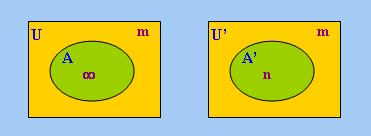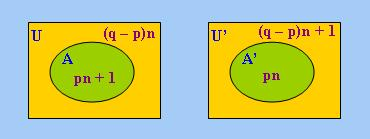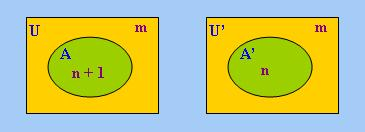「定理13」中的量詞都是<1>型量詞，但我們可以透過以下的「關係化」操作把<1>型量詞轉化為限定詞：

(Qrel)U(A)(B) ⇔ QA(A ∩ B)     (11)

(Q0)rel = (infinitely many)
(p / q)rel = (more than p / q)
(QR)rel = most
(Qeven)rel = (an even number of)

<1>型量詞及其「關係化」在「表達力」的強弱上有以下關係：

 Q(A) ⇔ Qrel(U)(A) ⇔ Qrelx (x = x, A(x))

(more than p / q)(A)(B) ⇔ ~(all but at most p / q)(A)(~B)

### 5.4 一階(量詞)邏輯的層級

 定理16： (i) I ~∈ FO(Q0)-D (ii) most ~∈ FO(QR)-D (iii) Q0 ~∈ FO(most)-D；Q0 ~∈ FO(Qeven)-D (iv) QR ~∈ FO(I)-D；QR ~∈ FO(Qeven)-D (v) Qeven ~∈ FO(MO)-D

FO(Qrel) ≤ FO(Q)     (12)

(infinitely many)(A)(B) ⇔ Q0x (A(x) ∧ B(x))
(an even number of)(A)(B) ⇔ Qevenx (A(x) ∧ B(x))
every(A)(B) ⇔ ∀x (A(x) ⇒ B(x))

FO(infinitely many) ≡ FO(Q0)
FO(an even number of) ≡ FO(Qeven)
FO(every) ≡ FO(∀) ≡ FO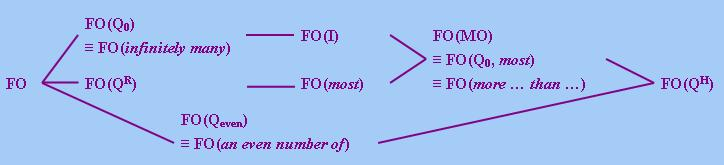## 6. 與單調量詞相關的可定義性

### 6.1 遞增量詞

(Qf)U(A) ⇔ |A| ≥ f(|U|)     (13)

 ((Q'f)rel)U(A)(B) ⇔ (Q'f)A(A ∩ B) (根據(11)) ⇔ |A ∩ B| ≥ f(|A|) (根據(13))     (14)

MON↑ ∪ MON↓ ⊆ BO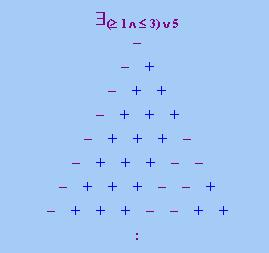Vaananen在Unary Quantifiers on Finite Models一文中提出以下定理(證明從略)：

(≥ 1 ∧ ≤ 3) ∨ > 5(A) ⇔ (Qfx (A(x)) ∧ ~Qgx (A(x))) ∨ Qhx (A(x))

 f(n) = 1，若n ∈ S 2，若n ~∈ S

 Q(A)(B) ⇔ |A ∩ B| ∈ S ⇔ 1 ≥ f(|A ∩ B|) (根據f的定義) ⇔ (A ∩ B = Φ) ∨ ∃x (x ∈ A ∩ B ∧ |{x}| ≥ f(|A ∩ B|)) ⇔ (A ∩ B = Φ) ∨ ∃x (x ∈ A ∩ B ∧ Qfrel(A ∩ B)({x})) (根據(14)) ⇔ ~∃x (A(x) ∧ B(x)) ∨ ∃x (A(x) ∧ B(x) ∧ Qfrely (A(y) ∧ B(y), y = x))

c([x, y]) = [c(x), c(y)]

 c(n) = r，若n為偶數 (15) g，若n為奇數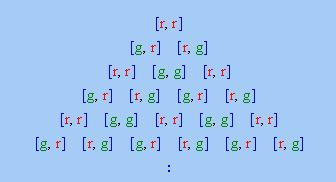BO ⊆ BCO

c(n) = r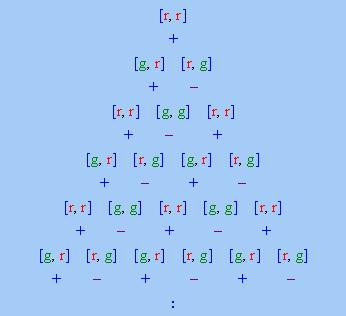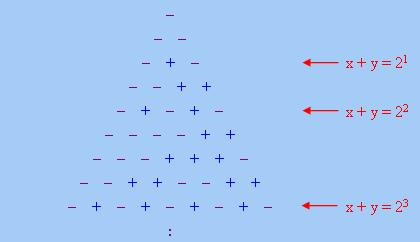### 6.2 光滑量詞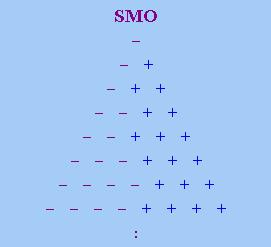F(n) ≤ F(n + 1) ≤ F(n) + 1     (16)

(some or none of an even number of)(A)(B) ⇔ |A|為偶數